## Chapter 4 triangles R.D. Sharma Solutions for Class 10th Math Exercise 4.7

Exercise 4.7

1. If the sides of a triangle are 3 cm, 4 cm, and 6 cm long, determine whether the triangle is a right-angled triangle.

Solution

Side of traingles are:
AB = 3cm
BC = 4 cm
AC = 6 cm
Now, we know that the triangle can be right angled if it follows the pythagoras theorem.
∴ AB2 = 32 = 9
BC2 = 42 = 16
AC2 = 62 = 36
Since, AB2 + BC2 ≠ AC2
Then, by converse of Pythagoras theorem, Triangle is not a right angled.

2. The sides of certain triangles are given below. Determine which of them right triangles are.
(i) a = 7 cm, b = 24 cm and c = 25 cm
(ii) a = 9 cm, b = l6 cm and c = 18 cm
(iii) a = 1.6 cm, b = 3.8 cm and c = 4 cm
(iv) a = 8 cm, b = 10 cm and c = 6 cm

Solution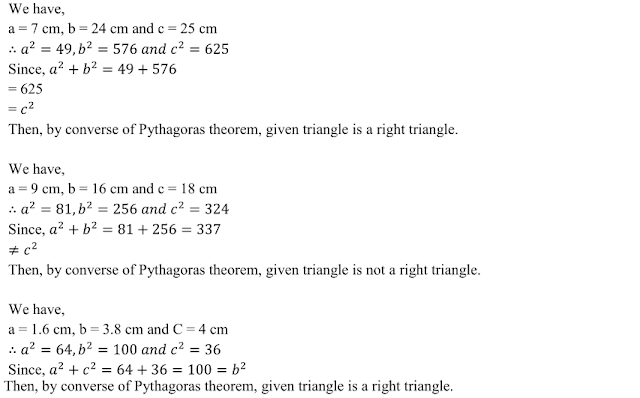3. A man goes 15 metres due west and then 8 metres due north. How far is he from the starting point ?

Solution4. A ladder 17 m long reaches a window of a building 15 m above the ground. Find the distance of the foot of the ladder from the building .

Solution5. Two poles of heights 6 m and 11 m stand on a plane ground. If the distance between their feet is 12 m, find the distance between their tops .

Solution6. In an isosceles triangle ABC, AB = AC = 25 cm, BC = 14 cm. Calculate the altitude from A on BC.

Solution7. The foot of a ladder is 6 m away from a wall and its top reaches a window 8 m above the ground. If the ladder is shifted in such a way that its foot is 8 m away from the wall, to what height does its tip reach ?

Solution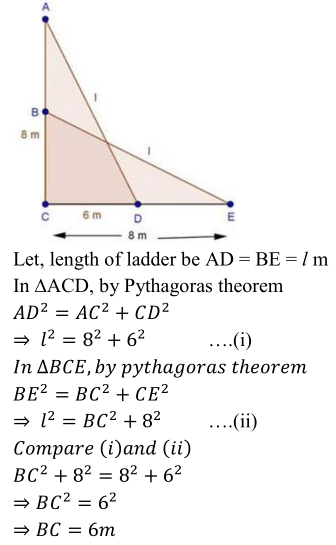8. Two poles of height 9 m and 14 m stand on a plane ground. If the distance between their feet is 12 m, find the distance between their tops.

Solution9. Using Pythagoras theorem determine the length of AD in terms of b and c shown in Fig. 4.221.Solution10. A triangle has sides 5 cm, 12 cm and 13 cm. Find the length to one decimal place, of the perpendicular from the opposite vertex to the side whose length is 13 cm.

Solution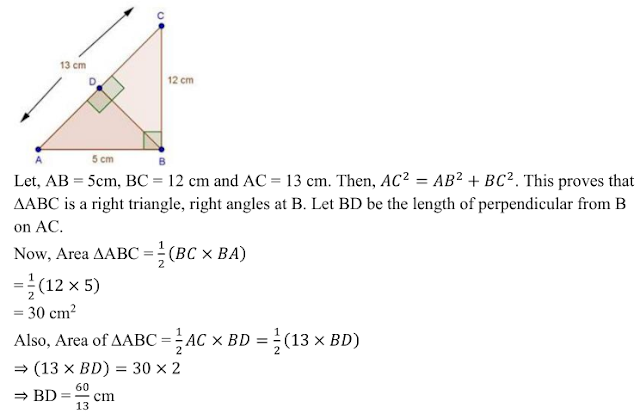11. ABCD is a square. F is the mid-point of AB. BE is one third of BC. If the area of ∆FBE =
108 cm2 , find the length of AC.

Solution12. In an isosceles triangle ABC, if AB = AC = 13 cm and the altitude from A on BC is 5 cm, find BC.

Solution13 . In a ∆ABC, AB = BC = CA = 2a and AD ⊥ BC. Prove that
(ii) Area (∆ABC) =√3a

Solution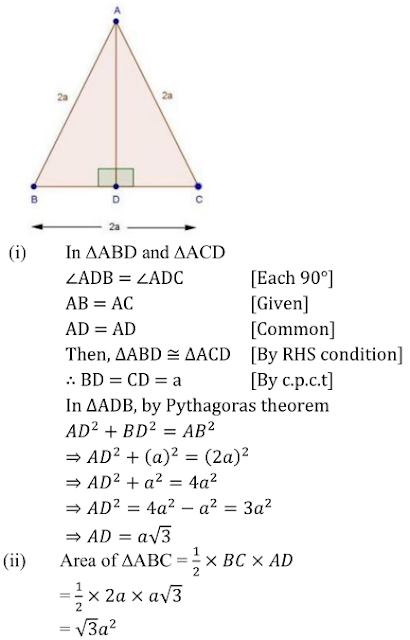14. The lengths of the diagonals of a rhombus are 24 cm and 10 cm. Find each side of the rhombus.

Solution15. Each side of a rhombus is 10 cm. If one of its diagonals is 16 cm find the length of the other diagonal.

Solution16. Calculate the height of an equilateral triangle each of whose sides measures 12 cm.

Solution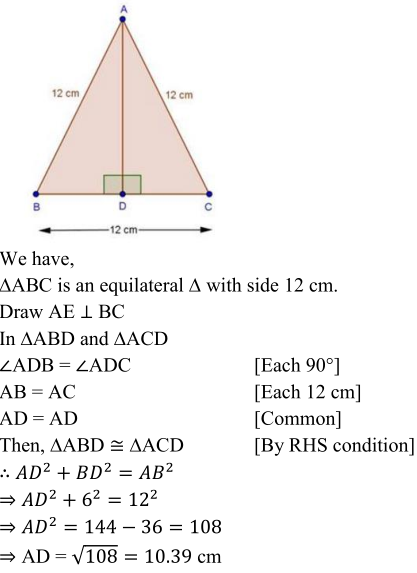17.  In Fig., 4.221, ∠B < 90° and segment AD ⊥ BC, show that
(i) b2 = h2 + a2 + x2 - 2ax
(ii) b2 = a2 + c2 - 2ax

Solution18. In an equilateral ∆ABC, AD ⊥ BC, prove that AD2 = 3BD2 .

Solution19. △ABD is a right triangle right angled at A and AC ⟂ BD. Show that:

(i) AB2 = CB × BD
(ii) AC2 = DC × BC
(iii) AD2 = BD × CD
(iv) AB2/AC2 = BD/DC

Solution(i)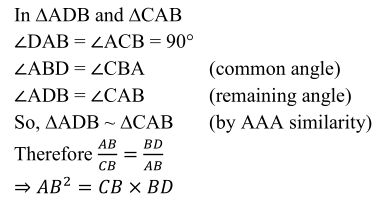(ii)(iii)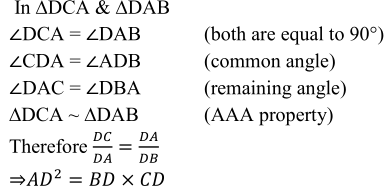(iv)20. A guy wire attached to a vertical pole of height 18 m is 24 m long and has a stake attached to the other end. How far from the base of the pole should the stake be driven so that the wire will be taut ?

Solution21. Determine whether the triangle having sides (a − 1) cm, 2√a cm and (a + 1) cm is a right - angled triangle.

Solution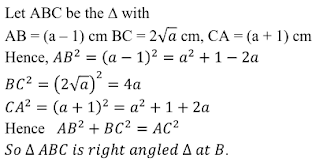22. In an acute-angled triangle, express a median in terms of its sides.

Solution23. In right-angled triangle ABC in which ∠C = 90°, if D is the mid-point of BC, prove that AB2 = 4 AD2 - 3 AC2 .

Solution24. In Fig. 4.223, D is the mid-point of side BC and AE ⊥ BC. If BC = a, AC = b, AB = c, ED = x, AD = p and AE = h, prove that:
(i) b2 = p2+ax+a4
(ii) c2 = p2 - ax+a2/4
(iii) b2+c2 = 2p2 + a2/2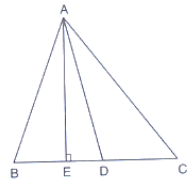Solution

(i)(ii)(iii)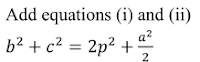25. In ∆ABC, ∠A is obtuse, PB ⊥AC and QC ⊥ AB. Prove that:
(i) AB × AQ = AC × AP
(ii) BC2 = (AC × CP + AB × BQ)

Solution26. In a right ∆ABC right-angled at C, if D is the mid-point of BC, prove that CD2 = 4(AD2 - AC2) .

Solution27. In a quadrilateral ABCD, ∠B = 90°, AD2 = AB2 + BC2 + CD2 , prove that ∠ACD = 90°.

Solution28. An aeroplane leaves an airport and flies due north at a speed of 1000km/hr. At the same time, another aeroplane leaves the same airport and flies due west at a speed of 1200 km/hr. How far apart will be the two planes after 1 hours ?

Solution